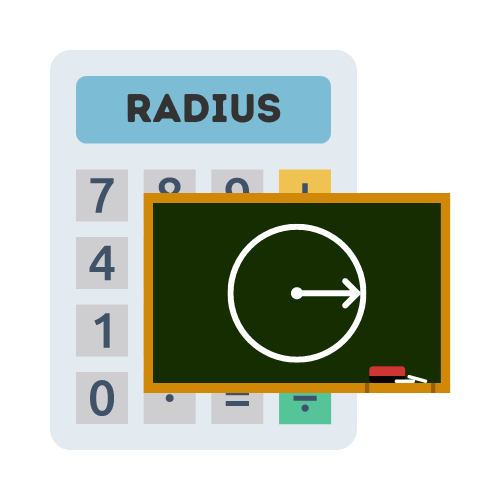# Radius of a Circle Calculator

## Calculate the radius of a circle with this free calculator

• Created by John Harris
• Reviewed by Ramesh Agarwal

The radius of the circle is:

## What is the radius of a circle?

The circle's radius can be described as the distance from the center of the circle to any point on the circumference of that circle (half a circle's width). It is an important property of a circle and its value can be found with the help of a simple formula.

## How to find the radius of a circle?

In the most cases, we should be able to find the radius from a circle fairly easily. It is the distance from the center of the circle to a point anywhere on the outer perimeter of the circle (also referred to as the semi perimeter of circle).

When we cannot find the radius, we can normally find the diameter instead. The diameter is the longest distance that passes through the circle, from one side to the other.

The radius of a circle calculation requires us to first know the surface area or volume of the circle. Once we have the area, we can then use that within out equation. We must divide the area by Pi (3.14), then get the square root of the result.

## Why do we calculate radius?

The main reasons why we calculate the radius is to use the value in other equations. Using the radius we can perform calculations to measure the circumference or the area of the circle. Knowing the radius also enables us to measure the diameter of the circle, giving a clearer picture of the dimensions of the circle.

## What is the formula for radius of a circle?

The formula for the radius of a circle is:

`Radius = (Area / 3.14)2`

The formula for radius can be carried out by taking the area of the circle and dividing it by pi (3.14) and then getting the square root.

You can use the radius of a circle when solving an equation where the radius is needed, such as finding the area of the circle, finding the circumference of the circle, or finding the average radius of a group of circles.

Obtaining the radius value is possible when you only have limited information about the measurements of the circle. If you know the surface area or volume of the circle, the value can be used to help determine the radius.

## FAQS

The radius of a circle is the distance from the center of the circle to any point on its circumference. It is denoted by the letter 'r'.

You can find the radius of a circle if you know the diameter by dividing the diameter by 2. The formula is r = d/2, where r is the radius and d is the diameter.

The circumference of a circle (C) is related to its radius (r) by the formula C = 2πr, where π is the mathematical constant pi (approximately 3.14159).

You can calculate the area of a circle if you know the radius using the formula A = πr², where A is the area and r is the radius.

No, the radius of a circle is a positive distance. It cannot be negative or zero.

The radius of a circle is usually measured in units such as centimeters (cm), meters (m), inches (in), or any other unit of length.